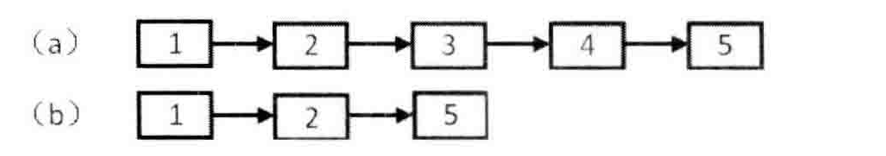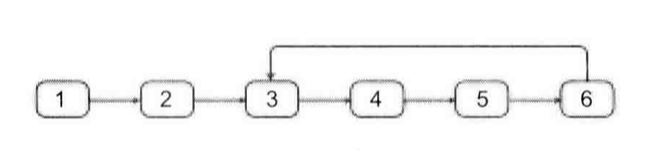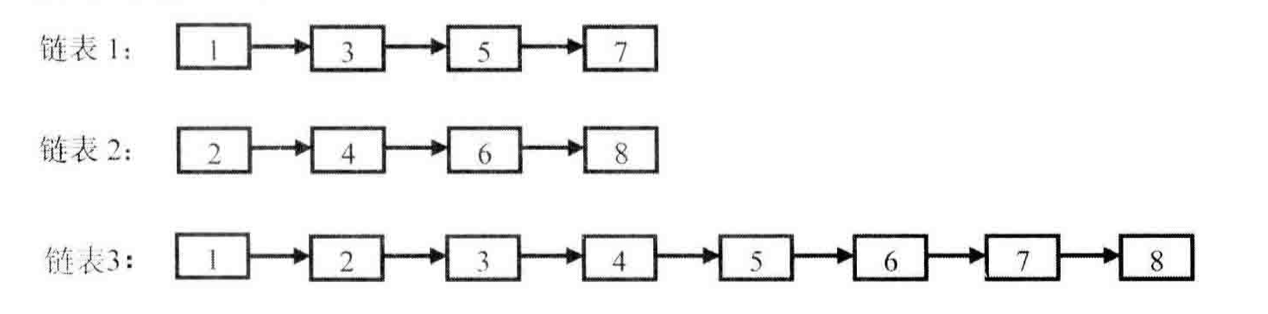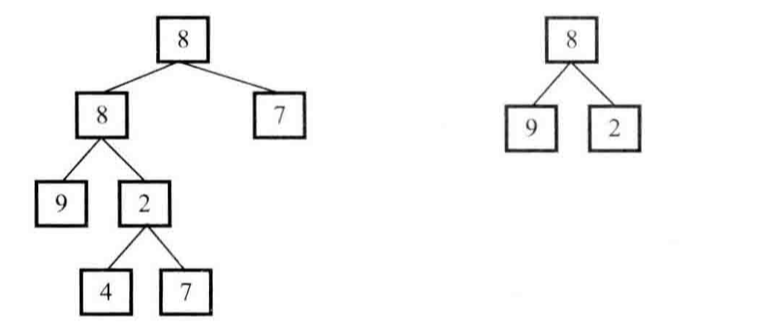# 高质量的代码

## 1. 代码的完整性¶

• 从三个方面确保代码的完整性
通常我们可以从功能测试、边界测试和负面测试3个方面设计测试用例

• 3种错误处理方式

1. 函数返回值
2. 设置全局变量
3. 抛异常

### 1.1 (16)数值的整数次方¶

LC-50-Pow(x, n)类似。 不过LeetCode上需要注意n的溢出问题。

private boolean invalidInput;

private double power(double base, int exponent) {
invalidInput = false;

if (exponent < 0 && base == 0) {
invalidInput = true;
return 0;
}

long absExponent = Math.abs(exponent * 1L);

double result = powerInner(base, absExponent);

return exponent < 0 ? 1.0 / result : result;
}

private double powerInner(double base, long exponent) {
double result = 1.0;

for (int i = 1; i <= exponent; i++) {
result *= base;
}

return result;
}


a^n=\begin{cases} a^{n/2} \cdot a^{n/2}, & n为偶数 \\ a^{(n-1)/2} \cdot a^{(n-1)/2} \cdot a, & n为奇数 \end{cases}

private double powerInner(double base, long exponent) {
if (exponent == 0) {
return 1;
} else if (exponent == 1) {
return base;
}

double result = powerInner(base, exponent >> 1);
result *= result;
if ((exponent & 0x1) == 1) {
result *= base;
}

return result;
}


### 1.2 (17)打印1到最大的n位数¶

private void print1ToMaxOfNDigits_1(int n) {
if (n <= 0) return;

char[] number = new char[n];

// 初始化字符串
for (int i = 0; i < n; i++) {
number[i] = '0';
}

while (!increment(number)) {
printNumber(number);
}
}

private boolean increment(char[] number) {
boolean isOverflow = false;
int takeOver = 0;
int length = number.length;

for (int i = length - 1; i >= 0; i--) {
int sum = number[i] - '0' + takeOver;
if (i == length - 1) {
sum++;
}

if (sum >= 10) {
// 累加有进位
if (i == 0) {
// 已经打印完毕了，设置为true，不在继续计数
isOverflow = true;
} else {
// 还没有打印完毕，在后续循环中进行进位操作
sum -= 10;
takeOver = 1;
number[i] = (char) (sum + '0');
}
} else {
// 累加没有进位，直接结束循环
number[i] = (char) ('0' + sum);
break;
}
}

return isOverflow;
}

// ====================公共函数====================
private void printNumber(char[] number) {
boolean begin0 = true;
for (char ch: number) {
// 只需要略过打头的0
if (begin0 && ch != '0') {
begin0 = false;
}
if (!begin0) {
System.out.print(ch);
}
}

System.out.println();
}


private void print1ToMaxOfNDigits_2(int n) {
if (n <= 0) return;

char[] number = new char[n];

// 设置第0位
for (int i = 0; i < 10; i++) {
number = (char) (i + '0');
print1ToMaxOfNDigits_2_Recursively(number, n, 0);
}
}

private void print1ToMaxOfNDigits_2_Recursively(char[] number, int length, int index) {
// 已经设置了最后一位，直接打印即可
if (index == length - 1) {
printNumber(number);
return;
}

// 设置第index+1位
for (int i = 0; i < 10; i++) {
number[index + 1] = (char) (i + '0');
print1ToMaxOfNDigits_2_Recursively(number, length, index + 1);
}
}


### 1.3 (18)删除链表的节点¶

#### 1.3.1 在O(1)时间删除链表结点¶

private ListNode deleteNode(ListNode head, ListNode toBeDeleted) {
if (head == null || toBeDeleted == null) {
return null;
}

// 要删除的结点不是尾结点
if (toBeDeleted.next != null) {
toBeDeleted.value = toBeDeleted.next.value;
toBeDeleted.next = toBeDeleted.next.next;
} else if (head == toBeDeleted) {
// 链表只有一个结点，删除头结点（也是尾结点）
} else {
// 链表中有多个结点，删除尾结点
ListNode p = head;
while (p.next != toBeDeleted) {
p = p.next;
}
p.next = null;
}

}


#### 1.3.2 删除链表中重复的结点¶private ListNode deleteDuplication(ListNode head) {
if (head == null) {
return null;
}

// 创建一个头结点
ListNode rHead = new ListNode(0);

ListNode p, q;

while (p.next != null) {
q = p.next;
boolean duplicate = false;

while (q.next != null && q.value == q.next.value) {
duplicate = true;
q = q.next;
}

if (duplicate) {
p.next = q.next;
} else {
p = q;
}
}

}


### 1.4 (19)正则表达式匹配¶

• 若不是，那么本次只需要比较字符串中第一个字符和模式中的第一个字符是否相匹配。若匹配，那么字符串和模式都向后移动一个字符，然后匹配剩余的字符串和模式。若不匹配，直接返回false。
• 若是'*',那么有两种匹配方式：
1. "aab"、"c*a*b"方式
此时在模式上向后移动两个字符即可。
2. "aaa"、"a*a"方式
此时如果第一个字符可以匹配，则在字符串上向后移动一个字符，模式保持不变即可。

class Solution {
public boolean isMatch(String text, String pattern) {
if (pattern.isEmpty()) return text.isEmpty();
boolean first_match = (!text.isEmpty() &&
(pattern.charAt(0) == text.charAt(0) || pattern.charAt(0) == '.'));

if (pattern.length() >= 2 && pattern.charAt(1) == '*'){
return (isMatch(text, pattern.substring(2)) ||
(first_match && isMatch(text.substring(1), pattern)));
} else {
return first_match && isMatch(text.substring(1), pattern.substring(1));
}
}
}


### 1.5 (20)表示数值的字符串¶

private int index;

private boolean isNumeric(String str) {
if (str == null) {
return false;
}

return isNumeric(str.toCharArray());
}

private boolean isNumeric(char[] str) {
index = 0;
boolean numeric = scanInteger(str);

// 如果出现'.'，接下来是数字的小数部分
if (index < str.length && str[index] == '.') {
index++;

// 下面一行代码用||的原因：
// 1. 小数可以没有整数部分，例如.123等于0.123；此时原来的numeric=false
// 2. 小数点后面可以没有数字，例如233.等于233.0；此时原来的numeric=true
// 3. 当然小数点前面和后面可以有数字，例如233.666；此时原来的numeric=true
numeric = scanUnsignedInteger(str) || numeric;
}

// 如果出现'e'或者'E'，接下来跟着的是数字的指数部分
if (index < str.length && (str[index] == 'e' || str[index] == 'E')) {
index++;

// 下面一行代码用&&的原因：
// 1. 当e或E前面没有数字时，整个字符串不能表示数字，例如.e1、e1；此时原来的numeric=false
// 2. 当e或E后面没有整数时，整个字符串不能表示数字，例如12e、12e+5.4；此时原来的numeric=true
numeric = numeric && scanInteger(str);
}

return index == str.length && numeric;
}

private boolean scanInteger(char[] str) {
if (index < str.length && (str[index] == '-' || str[index] == '+')) {
index++;
}
return scanUnsignedInteger(str);
}

private boolean scanUnsignedInteger(char[] str) {
int tmp = index;

while (index < str.length && str[index] >= '0' && str[index] <= '9') {
index++;
}

return index > tmp;
}


### 1.6 (21)调整数组顺序使奇数位于偶数前面¶

void reorderOddEven_1(int[] pData, int length) {
if (pData == null || length == 0) {
return;
}

int lo = 0, hi = length - 1;

while (lo < hi) {
// 向后移动lo，直到它指向偶数
while (lo < hi && (pData[lo] & 0x1) != 0) {
lo++;
}

// 向前移动hi，直到它指向奇数
while (lo < hi && (pData[hi] & 0x1) == 0) {
hi--;
}

if (lo < hi) {
int temp = pData[lo];
pData[lo] = pData[hi];
pData[hi] = temp;
}
}
}


/** A function that takes 1 argument. */
private interface Function<P1, R> {
R invoke(P1 n);
}

private void reorderOddEven_2(int[] pData, int length) {
reorder(pData, length, new IsEvenFunction());
}

private void reorder(int[] pData, int length, Function<Integer, Boolean> function) {
if (pData == null || length == 0) {
return;
}

int lo = 0, hi = length - 1;

while (lo < hi) {
// 向后移动pBegin，直到它指向偶数
while (lo < hi && !function.invoke(pData[lo])) {
lo++;
}

// 向前移动pEnd，直到它指向奇数
while (lo < hi && function.invoke(pData[hi])) {
hi--;
}

if (lo < hi) {
int temp = pData[lo];
pData[lo] = pData[hi];
pData[hi] = temp;
}
}
}

private class IsEvenFunction implements Function<Integer, Boolean> {
@Override
public Boolean invoke(Integer n) {
return (n & 1) == 0;
}
}


## 2. 代码的鲁棒性¶

### 2.1 (22)链表中倒数第k个结点¶

private ListNode findKthToTail(ListNode pListHead, int k) {
if (pListHead == null || k <= 0) {
return null;
}

ListNode p = pListHead;
int i = 1;
while (i < k && p != null) {
p = p.next;
i++;
}

if (p == null) {
return null;
}

ListNode q = pListHead;
while (p.next != null) {
p = p.next;
q = q.next;
}

return q;
}


### 2.2 (23)链表中环的入口结点¶1. 找出环中任意一个节点；
2. 得到环中节点的数目；
3. 找到环的入口节点。
private ListNode entryNodeOfLoop(ListNode head) {
ListNode meetingNode = meetingNode(head);
if (meetingNode == null) {
return null;
}

// 计算环中节点个数
int nodesInLoop = 1;
ListNode node1 = meetingNode;
while (node1.next != meetingNode) {
nodesInLoop++;
node1 = node1.next;
}

// 将node1从头开始移动nodesInLoop次
for (int i = 0; i < nodesInLoop; i++) {
node1 = node1.next;
}

// 将node1、node2同时移动
ListNode node2 = head;
while (node1 != node2) {
node1 = node1.next;
node2 = node2.next;
}

return node1;
}

private ListNode meetingNode(ListNode head) {
if (head == null) {
return null;
}

ListNode slow = head.next;
if (slow == null) {
return null;
}

ListNode fast = slow.next;
while (fast != null && slow != null) {
if (fast == slow) {
return fast;
}

slow = slow.next;

fast = fast.next;
if (fast != null) {
fast = fast.next;
}
}

return null;
}


### 2.3 (24)反转链表¶

private ListNode reverseList(ListNode head) {
if (head == null || head.next == null) {
}

ListNode p = head, q = p.next;

while (q != null) {
p = q.next;
q = p;
}

}


• 输入的链表头指针是null
• 输入的链表只有一个节点
• 输入的链表有多个节点

private ListNode reverseList(ListNode head) {
if (head == null || head.next == null) {
}

ListNode next = head.next;
ListNode reversed = reverseList(next);

return reversed;
}


### 2.4 (25)合并两个排序的链表¶class Solution {
public ListNode mergeTwoLists(ListNode l1, ListNode l2) {
ListNode result = new ListNode(0), p = l1, q = l2, m = result;

while (p != null || q != null) {
if (p == null) {
m.next = q;
return result.next;
}
if (q == null) {
m.next = p;
return result.next;
}
if (p.val < q.val) {
m.next = new ListNode(p.val);
p = p.next;
} else {
m.next = new ListNode(q.val);
q = q.next;
}
m = m.next;
}

return result.next;
}
}


### 2.5 (26)树的子结构¶• 第一步，在树A中找到和树B的根节点的值一样的节点R
• 第二步，判断树A中以R为根节点的子树是不是包含和树B一样的结构
private boolean hasSubtree(BinaryTreeNode root1, BinaryTreeNode root2) {
boolean result = false;

if (root1 != null && root2 != null) {
result = doesTree1HasTree2(root1, root2);
if (!result) {
result = hasSubtree(root1.left, root2);
}
if (!result) {
result = hasSubtree(root1.right, root2);
}
}

return result;
}

private boolean doesTree1HasTree2(BinaryTreeNode root1, BinaryTreeNode root2) {
if (root2 == null) {
return true;
}

if (root1 == null) {
return false;
}

if (root1.value != root2.value) {
return false;
}

return doesTree1HasTree2(root1.left, root2.left) &&
doesTree1HasTree2(root1.right, root2.right);
}


Tip